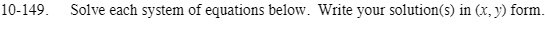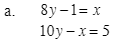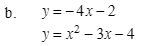### Home > CAAC > Chapter 10 > Lesson 10.4.3 > Problem10-149

10-149.You can use the substitution method.

10y − (8y − 1) = 5

Solve for y.

10 − 8y + 1 = 5
2y = 4
y = 2

Substitute y into one of the equations and solve for x.

8(2) − 1 = x
16 − 1 = x
x = 15

(15, 2)You can use the substitution method.

−4x − 2 = x2 − 3x − 4

Set the equation equal to zero.

0 = x2 − 3x + 4x − 4 + 2
x2 + x − 2 = 0

Solve for x.

Factor:
(x − 1)(x + 2) = 0
x − 1 = 0 x + 2 = 0
x = 1 x = −2

Substitute both x's into one of the equations to solve for y.

y = −4(1) − 2 y = −4(−2) − 2
y = −6 y = 6

(1, −6) (−2, 6)